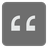Beginning Zeros
Elementary+
English RU

You have a string that consist only of digits. You need to find how many zero digits ("0") are at the beginning of the given string.

Input: A string, that consist of digits.

Output: An Int.

Example:

```beginning_zeros('100') == 0
beginning_zeros('001') == 2
beginning_zeros('100100') == 0
beginning_zeros('001001') == 2
beginning_zeros('012345679') == 1
beginning_zeros('0000') == 4
```

Precondition: 0-9